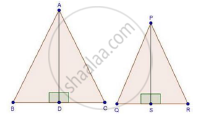Share
Notifications

View all notifications
Books Shortlist
Your shortlist is empty

# Solution for The Areas of Two Similar Triangles Are 100 Cm2 and 49 Cm2 Respectively. If the Altitude the Bigger Triangle is 5 Cm, Find the Corresponding Altitude of the Other. - CBSE Class 10 - Mathematics

Login
Create free account

Forgot password?

#### Question

The areas of two similar triangles are 100 cm2 and 49 cm2 respectively. If the altitude the bigger triangle is 5 cm, find the corresponding altitude of the other.

#### SolutionWe have, ΔABC ~ ΔPQR

Area(ΔABC) = 100 cm2,

Area (ΔPQR) = 49 cm2

AD = 5 cm

And AD and PS are the altitudes

By area of similar triangle theorem

("Area"(triangleABC))/("Area"(trianglePQR))="AB"^2/"PQ"^2

rArr100/49="AB"^2/"PQ"^2

rArr10/7="AB"/"PQ"             ............(i)

In ΔABD and ΔPQS

∠B = ∠Q                               [ΔABC ~ ΔPQR]

∠ADB = ∠PSQ                      [Each 90°]

Then, ΔABD ~ ΔPQS            [By AA similarity]

therefore"AB"/"PQ"="AD"/"PS"      .........(ii)[Corresponding parts of similar Δ are proportional]

Compare (i) and (ii)

"AD"/"PS"=10/7

rArr5/"PS"=10/7

rArr"PS"=(5xx7)/10=3.5 cm

Is there an error in this question or solution?

#### Video TutorialsVIEW ALL 

Solution The Areas of Two Similar Triangles Are 100 Cm2 and 49 Cm2 Respectively. If the Altitude the Bigger Triangle is 5 Cm, Find the Corresponding Altitude of the Other. Concept: Areas of Similar Triangles.
S